Courses

# Test: Wave Optics (CBSE Level With Solutions)

## 30 Questions MCQ Test Physics Class 12 | Test: Wave Optics (CBSE Level With Solutions)

Description
This mock test of Test: Wave Optics (CBSE Level With Solutions) for Class 12 helps you for every Class 12 entrance exam. This contains 30 Multiple Choice Questions for Class 12 Test: Wave Optics (CBSE Level With Solutions) (mcq) to study with solutions a complete question bank. The solved questions answers in this Test: Wave Optics (CBSE Level With Solutions) quiz give you a good mix of easy questions and tough questions. Class 12 students definitely take this Test: Wave Optics (CBSE Level With Solutions) exercise for a better result in the exam. You can find other Test: Wave Optics (CBSE Level With Solutions) extra questions, long questions & short questions for Class 12 on EduRev as well by searching above.
QUESTION: 1

### Is light a particle or a wave?

Solution:

Explanation:explanation none

QUESTION: 2

### Referring to the Young’s double slit experiment, Phase difference corresponding to a Path Difference of λ /3 is

Solution:

We know that,
Phase difference=K× path difference. K=2π/ λ.
Therefore, phase difference=(2π/ λ) ×( λ/3)=2π/3=120 degree.

QUESTION: 3

### The angle of incidence at which the reflected beam is fully polarized is called

Solution:

Brewster's angle is an angle of incidence at which light with a particular polarization is perfectly transmitted through a transparent dielectric surface, with no reflection. When unpolarized light is incident at this angle, the light that is reflected from the surface is therefore perfectly polarized.

QUESTION: 4

The refractive index of glass is 1.5 which of the two colors red and violet travels slower in a glass prism?

Solution:

(a) Speed of light in glass is c/μ=2×108m/s

(b) The speed of light in glass is not independent of the colour of light. The refractive index of a violet component of white light is greater than the refractive index of a red component. Hence, the speed of violet light is less than the speed of red light in glass. Hence, violet light travels slower than red light in a glass prism.

QUESTION: 5

In a young’s double slit experiment, the central bright fringe can be identified by

Solution:

When we use white light, the central bright will have light from all wavelengths as none of them cancel out. Hence the central bright fringe appears white.
For other bright fringes, depending on the wavelength of light constructive interference will not take place for certain wavelengths. Hence they will not be white, rather will be coloured, hence differentiated from central fringe.

QUESTION: 6

The propagation of light is best described by,

Solution:

Light as a wave: Light can be described (modeled) as an electromagnetic wave. In this model, a changing electric field creates a changing magnetic field. This changing magnetic field then creates a changing electric field and BOOM - you have light. Unlike many other waves (sound, water waves, waves in a football stadium), light does not need a medium to “wave” in.

QUESTION: 7

In general the term diffraction is used

Solution:

The process by which a beam of light or other system of waves is spread out as a result of passing through a narrow aperture or across an edge, typically accompanied by interference between the waveforms produced.

QUESTION: 8

Shape of the wave front of light diverging from a point source is

Solution:

The shape of the wave front in case of a light diverging from a point source is spherical and is shown in the given figure. The shape of the wavefront in case of a light emerging out of a convex lens when a point source is placed at its focus is a parallel grid.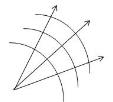QUESTION: 9

Emission and absorption is best described by,

Solution:

Distance between the slits, d=0.28×10−3 m
Distance between the slits and the screen, D=1.4m
Distance between the central fringe and the fourth (n=4) fringe,
u=1.2×10−2 m
In case of a constructive interference, we have the relation for the distance between the two fringes as:
u=nλD/d
⇒λ=ud/nD=6×10−7m=600nm

QUESTION: 10

In a Young’s double-slit experiment, the slits are separated by 0.28 mm and the screen is placed 1.4 m away. The distance between the central bright fringe and the fourth bright fringe is measured to be 1.2 cm. Determine the wavelength of light used in the experiment

Solution:

Both emission and absorption techniques can be used to get the same information about the energy levels of an atom. The dark lines correspond to the frequencies of light that have been absorbed by the gas. As the photons of light are absorbed by electrons, the electrons move into higher energy levels.

QUESTION: 11

Diffraction also refers to

Solution:

Diffraction is defined as the process by which a beam of light or other system of waves is spread out as a result of passing through a narrow aperture or across an edge, typically accompanied by interference between the waveforms produced.

QUESTION: 12

Shape of the wave front of light emerging out of a convex lens when a point source is placed at its focus.

Solution:

Plane. The shape of the wavefront in case of a light emerging out of a convex lens when a point source is placed at its focus is a parallel grid.

QUESTION: 13

A beam of light consisting of two wavelengths, 650 nm and 520 nm, is used to obtain interference fringes in a Young’s double-slit experiment. Find the distance of the third bright fringe on the screen from the central maximum for wavelength 650 nm.

Solution:

Solution :- Let at liner distance 'y' from center of screen the bright fringes due to both wavelength coincides. Let the n1 number of bright fringe with wevelength λ1 coincides with n2 number of bright fringe with wavelength λ2

We can write

y = n1​β1 = n2β2

n1 = λ1D/d                = n2​Dλ2/d                 or n1λ1=n2λ2

​Also at first position of coincide the th bright fringe of one will coincide with (n+1)th bright fringe of other.

If λ2<λ1,

So, then n2>n1

and n2 = n1+1

Using equation (ii) in equation (i)

n1λ1=(n1+1)λ2

​n1(650)×10^−9 = (n1+1)520×10^−9

65n1 = 52n1 + 52 or 12n1 = 52or n1=3

Thus, y = n1β1 = 3[(6.5×10^−7)(1.2)]/2×10^−3 = 1.17×10^−.3

m=1.17mm

QUESTION: 14

A beam of light has a wavelength of 650 nm in vacuum. What is the wavelength of these waves in the liquid whose index of refraction at this wavelength is 1.47?

Solution:

We know that,
Wavelength of light in a material:
λ= λ0/n
where, λ=wavelength of material, λ0=wavelength of light in vacuum,
n=index of refraction of material.
So, 650x10-9m/1.47=442.177nm.

QUESTION: 15

wave front is

Solution:
QUESTION: 16

A beam of light consisting of two wavelengths, 650 nm and 520 nm, is used to obtain interference fringes in a Young’s double-slit experiment. What is the least distance from the central maximum where the bright fringes due to both the wavelengths coincide?

Solution:

Fringe width of 650nm wavelength is 650D/d and that of 520nm wavelength is 520D/d
Where D is distance between slits and screen and d is distance between screens.
The common constructive pattern will be seen at the minimum possible common multiple of both the wavelengths.

QUESTION: 17

A parallel beam of light in air makes an angle of 47.5 with the surface of a glass plate having a refractive index of 1.66. What is the angle between the reflected part of the beam and the surface of the glass?

Solution:

The angle of incidence = 90 - 47.5 = 42.5
Now by snells’ laws we get
sin r = sin 42.5 / 1.66
Thus we get r = 24.01
Thus the angle with surface = 90 -24.01 = 65.99

QUESTION: 18

Shape of the wave front of portion of the wave front of light from a distant star intercepted by the Earth

Solution:

Plane (a small area on the surface of a large sphere is nearly planar). The portion of the wavefront of light from a distant star intercepted by the Earth is a plane.

QUESTION: 19

Which of the following phenomenon cannot be explained by diffraction?

Solution:

The bright colors seen in an oil slick floating on water or in a sunlit soap bubble are caused by interference. The brightest colors are those that interfere constructively. This interference is between light reflected from different surfaces of a thin film; thus, the effect is known as thin film interference.

QUESTION: 20

According to Huygens principle

Solution:
Every point on a wave-front may be considered a source of secondary spherical wavelets which spread out in the forward direction at the speed of light. The new wave-front is the tangential surface to all of these secondary wavelets.

According to Huygens' principle, a plane light wave propagates though free space at the speed of light, c. The light rays associated with this wave-front propagate in straight-lines. It is also fairly straightforward to account for the laws of reflection and refraction using Huygens' principle.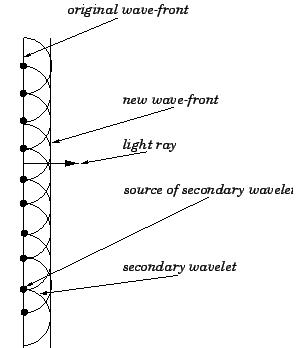QUESTION: 21

For distances much greater than Fresnel distance ZF

Solution:
QUESTION: 22

Estimate the distance for which ray optics is good approximation for an aperture of 4 mm and wavelength 400 nm.

Solution:

Here,
Aperture, a = 4 mm = 4 × 10-3 m
Wavelength, λ = 400 nm = 400 × 10-9 m = 4 × 10-7 m

Ray optics is good approximation upto distances equal to Fresnel's distance (ZF).
Fresnel's distance is given by,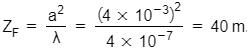QUESTION: 23

The critical angle for total internal reflection at a liquid–air interface is 42.5 If a ray of light traveling in air has an angle of incidence at the interface of 35 what angle does the refracted ray in the liquid make with the normal?

Solution:

From air to liquid:
1 ->air
2->liquid

n1sinθ1= n2sinθ2
1 x sin35o=1.48sinθ2
θ2=arcsin((1xsin35o)/1.48)=22.8o

QUESTION: 24

According to Huygens construction relation between old and new wave fronts is

Solution:

The Huygens-Fresnel principle states that every point on a wavefront is a source of wavelets. These wavelets spread out in the forward direction, at the same speed as the source wave. The new wavefront is a line tangent to all of the wavelets.
The correct answer is option B.

QUESTION: 25

We say that a light wave is linearly polarized in y direction if

Solution:
QUESTION: 26

A parallel beam of light of wavelength 500 nm falls on a narrow slit and the resulting diffraction pattern is observed on a screen 1 m away. It is observed that the first minimum is at a distance of 2.5 mm from the centre of the screen. Find the width of the slit.

Solution:

Wavelength of light beam, λ

Distance of the screen from the slit, D=1m

For first minima, n=1

Distance between the slits is d

Distance of the first minimum from the centre of the screen can be obtained as, x = 2.5mm = 2.5×10−3

Now, nλ = xd/D

⇒ d= nλD/x = 0.2mm

Therefore, the width of the slits is 0.2 mm.

QUESTION: 27

Relation between ray and wave front is

Solution:

A wave front is a line representing all parts of a wave that are in phase and an equal number of wavelengths from the source of the wave. A ray is a line extending outward from the source and representing the direction of propagation of the wave at any point along it. Rays are perpendicular to wave fronts.

QUESTION: 28

Two plane mirrors intersect at right angles. A laser beam strikes the first of them at a point 11.5 cm from their point of intersection, as shown in figure For what angle of incidence at the first mirror will this ray strike the midpoint of the second mirror (which is 28.0 cm long) after reflecting from the first mirror?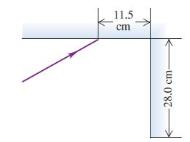Solution: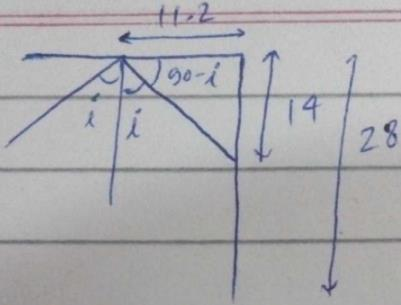Tan(90-i)=14/11.2
Cot i=14/11.2
I=cot-1(14/11.2)
I=39.4o

QUESTION: 29

Two sources of light are coherent if they have

Solution:

In physics, two wave sources are perfectly coherent if they have a constant phase difference and the same frequency (amplitude may be different).
As c be the speed of light which is constant.
Using, c=νλ
Now the same ν gives the same λ. for the two light sources.
Example: y1​=A1​sinwt and y2​=A2​sin(wt+ϕ) where ϕ is constant.

QUESTION: 30

If we have two coherent sources S1andS2 vibrating in phase, then for an arbitrary point P constructive interference is observed whenever the path difference is

Solution:

Constructive interference occurs when the path differences (S1​P−S2​P) is an integral multiple of λ or (S1​P−S2​P)=nλ
Where n=0,1 ,2 3,….